# 7.4 Control Costs

"The process of monitoring the status of the project to update project costs and managing changes to he cost baseline."

The definition shown above in italics is taken from the Glossary of the Project Management Institute, A Guide to the Project Management Body of Knowledge, (PMBOK® Guide) – Fifth Edition, Project Management Institute Inc., 2013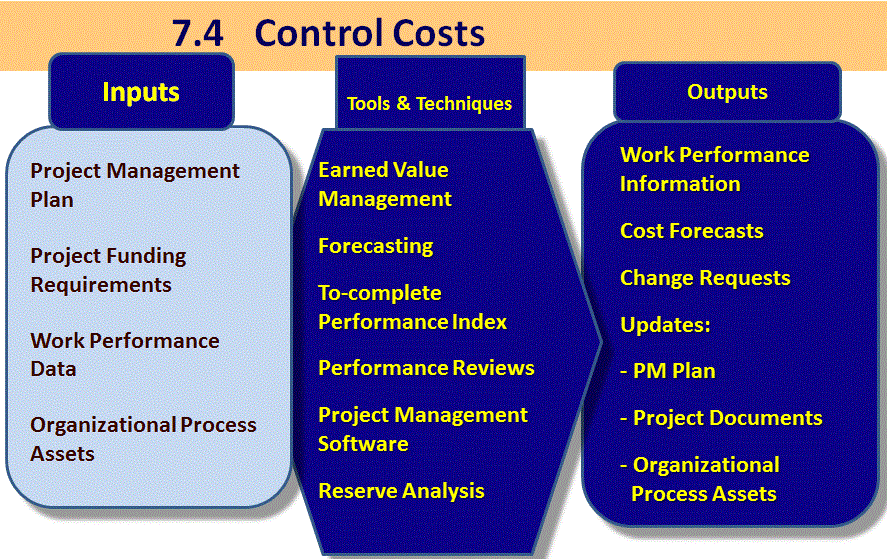Project Management Institute, A Guide to the Project Management Body of Knowledge, (PMBOK® Guide) – Fifth Edition, Project Management Institute Inc., 2013 Figure 7-10 Page 215

Cost Control

Change Control System for Cost

• Integrated with the Integrated Change Control Process
• Documented in the Cost Management Plan
• Any increase in the authorised budget can only be approved through 4.5 Perform Integrated Change Control process

Project cost control requires:

• Monitoring cost performance
• Ensuring that only appropriate project changes are included in a revised cost baseline
• Informing project stakeholders of authorized changes to the project that will affect costs

Earned Value Analysis (or Earned Value Management (EVM) is an important way to control costs

## Earned Value Management (EVM)

• Measures project progress as well as forecast cost at completion
• Compares actuals for schedule and cost performance against planned performance
• Quite important to understand the monetary value of work completed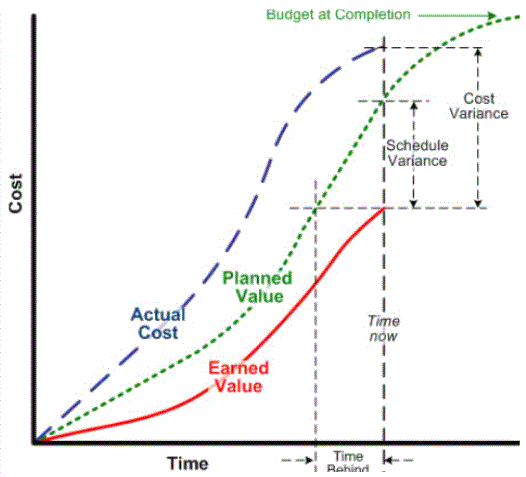• What percentage of the work is complete?
• Where is my money going?
• How much did we spend so far compared with what we budgeted (the forecast)?
• What percentage of the work is complete?
• Why are we behind schedule?
• What is your estimate at completion?

## Earned Value concepts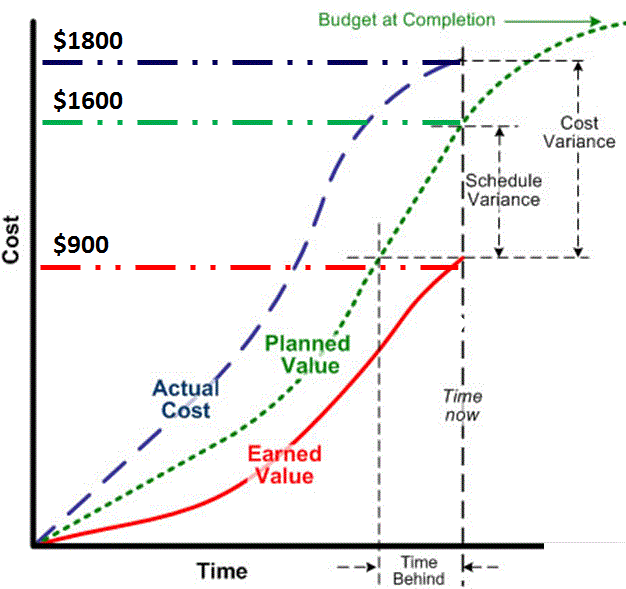Planned Value = the estimated cost of what we planned to do at report point

Total PV = BAC = PMB (Performance Measurement Baseline)

Earned Value = the estimated cost of the work that was done at report point = PV x progress

Actual Costs = Realised costs incurred for the work performed

Example:

If we were to look at the EV at point of measurement in the image below, this shows we are behind schedule.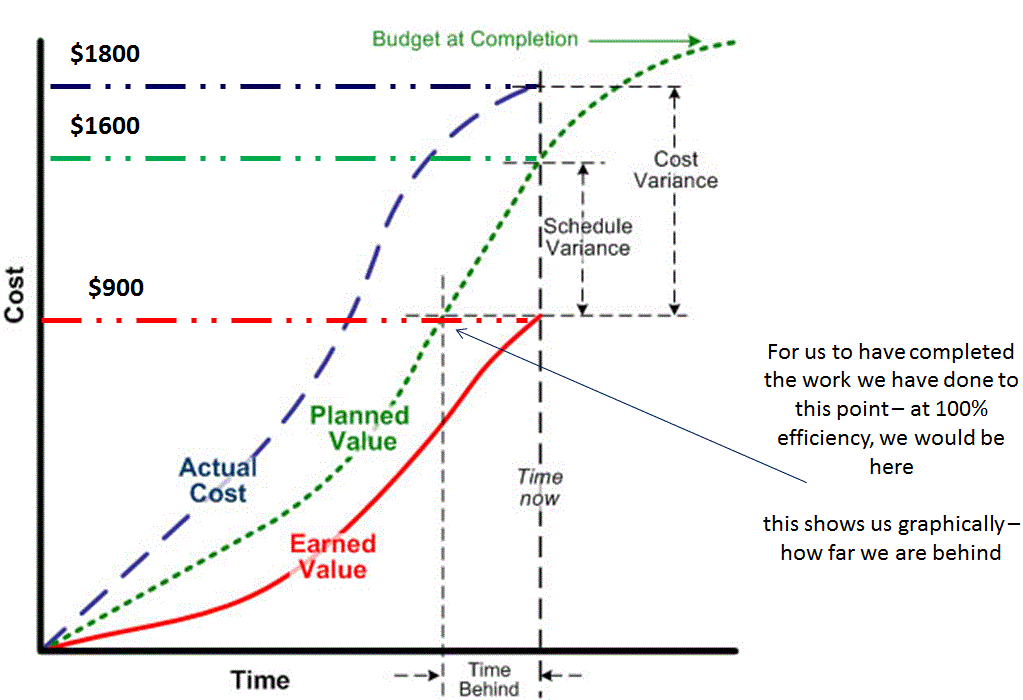## EVM measurements

 EVM Measures Equation Schedule variance SV = EV - PV Cost variance CV = EV - AC Schedule performance index SPI = EV / PV Cost performance index CPI = EV / AC

Cost variance - The difference between earned value and actual costs incurred.

• CV = EV – AC.
• - CV = The project is performing over budget.
• + CV = The project is performing below budget.
• 0 CV = The project is running on track

Example: Calculating schedule variance for a University Campus Build project.

• Total project budget is £425,800.
• £325,500 has to be spent by end of phase 3.
• Only £319,425 worth of work is completed.
• £319,425 (EV) - £325,500 (PV) = -£6,075 (SV) - project is behind schedule.

## What does this signify?

Example 1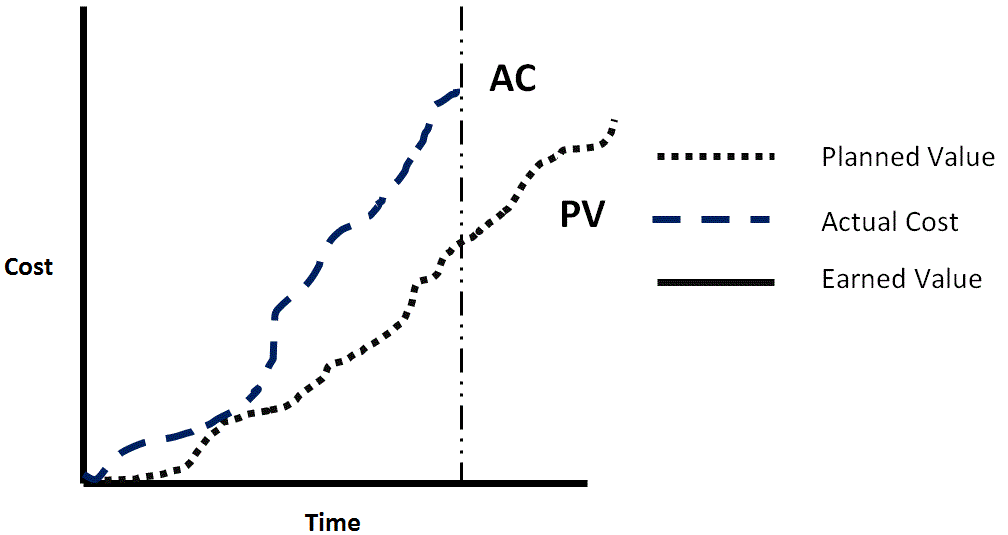Nothing, there is no earned value line and we cannot determine the project status

Example 2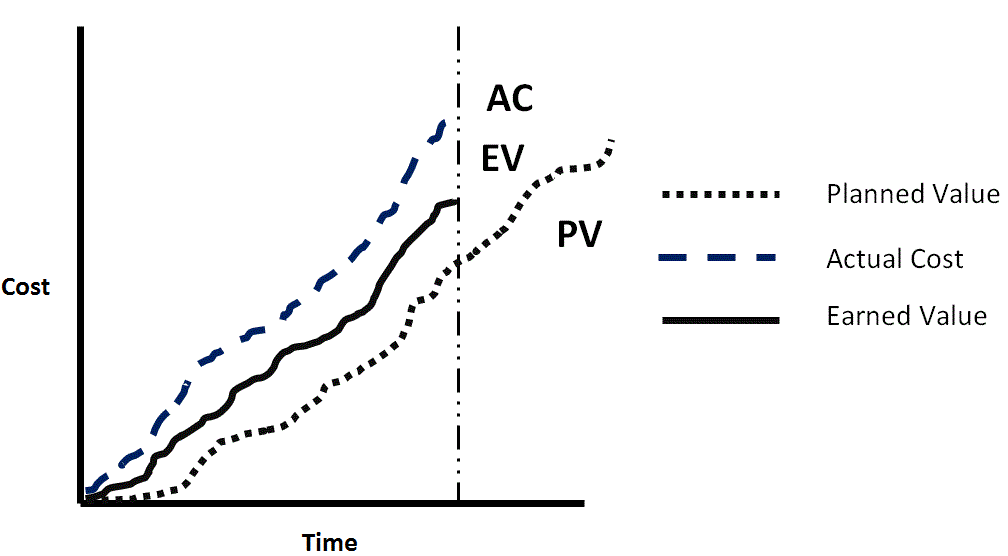• Time CV = -
• SV = +
• Over-achieving, but overspent for the achievement
• Money being wasted, e.g. unnecessary overtime

Example 3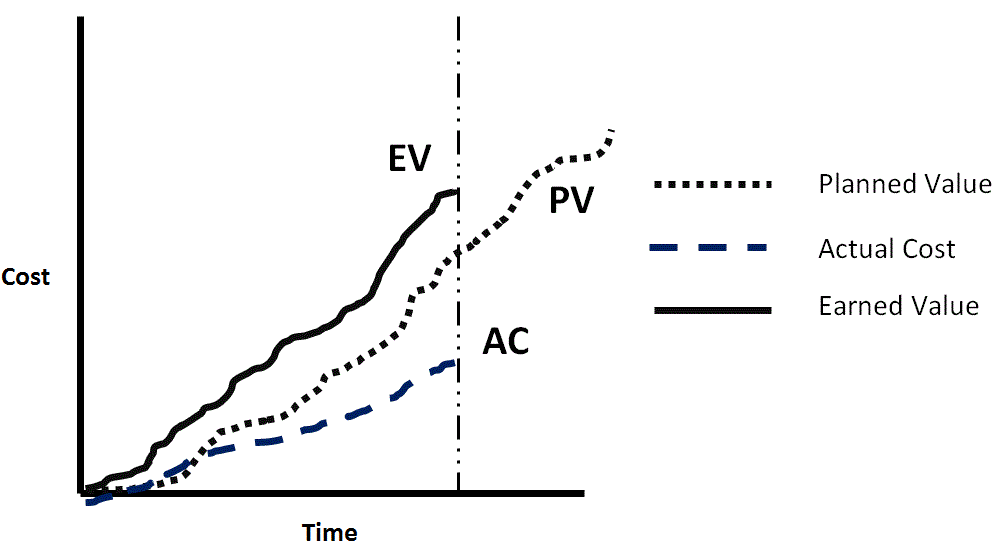• CV = +
• SV = +
• Over-achieving, and underspending
• No action needed (unless there is an issue with NOT spending the customers money)

Example 4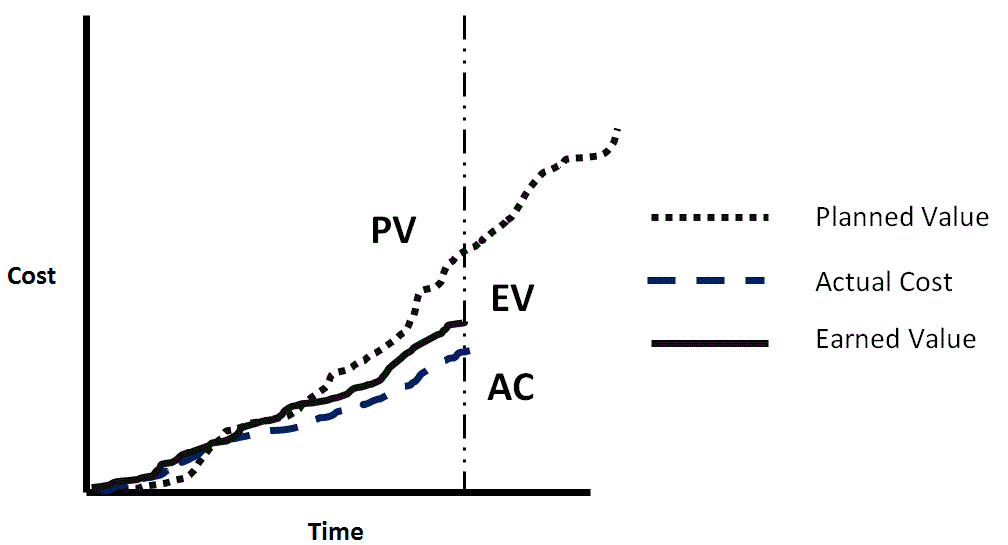• CV = +
• SV = -
• Under-achieving on the project, but overachieving for the spend
• Team performing well but under-resourced

Example 5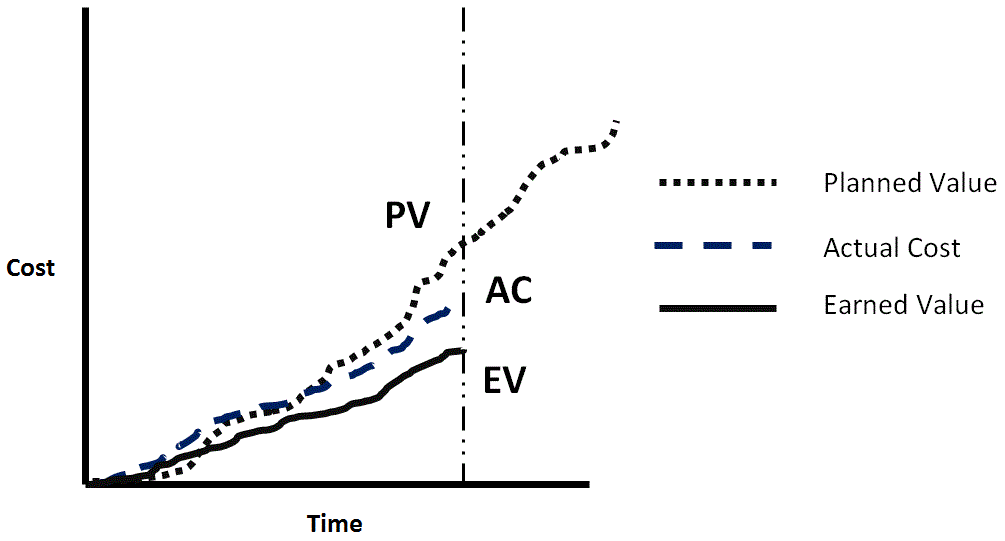• CV = -
• SV = -
• Under-achieving on the project & overspending for the work done
• Team under-resourced and under-performing

## Lets Have Some Fun

Our Project has:

• PV = £290,000
• EV = £260,000
• AC = £140,000

Formulae:

• SV = EV – PV
• CV = EV – AC
• SPI = EV/PV
• CPI = EV/AC

Work out:

• what this means for the project
• the SV
• the CV
• the SPI
• the CPI

## Visualizing EAC, ETC and VAC

EAC = as of today, how much do we expect the project to cost?

ETC = how much more do we estimate the project to cost from today?

VAC = the difference between original total costs and new forecast total costs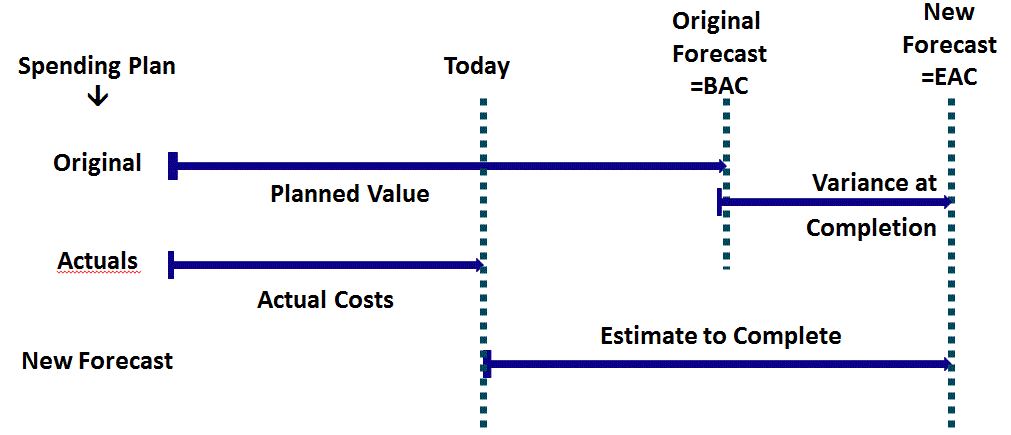Estimate At Completion

What do we currently forecast the total project to cost

4 formulae for different situations:

1. If we are 80% efficient and we don't know what the problem is, then we use this formula i.e. will carry on at 80% efficiency:

EAC = BAC / cumulative CPI = BAC/CPI

The word cumulative is used to denote the value at this point in time i.e. cumulative up to now.

2. If we are 80% efficient and we know what the problem is then we return to the budgeted rate and use this formula

EAC = AC + (BAC – EV)

3. If past assumptions are faulty then the PM or project team have no confidence in the original estimate for remaining work

EAC = AC + new bottom up estimate

4. This is used in special cases; considers both SPI and CPI.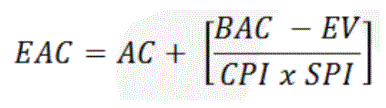There is an assumption that the project is over budget (negative cost performance) and the schedule has a completion deadline

ETC - Estimate to Complete is

Based on actual costs of work performed to date, need to calculate cost of work remaining

If things have not been going to plan (behind schedule and over budget) will/should the actual costs be used?

This formula covers all of the 4 EAC situations:

• ETC = EAC-AC

VAC - Variance at Completion is:

How different will the outturn budget be from the original forecast

• VAC = BAC - EAC

## To-Complete Performance Index (TCPI)

What level of efficiency is required to be obtained, from the resources, for the remainder of the project

The performance level we should use from this point to get the project back to BAC (or EAC):

• TCPI = Remaining work / Remaining project budget.
• TCPI (BAC) =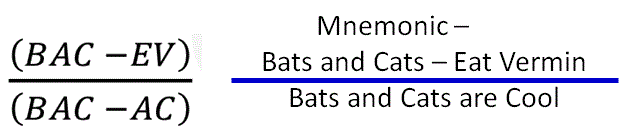• TCPI (EAC) = (BAC – EV) / (EAC – AC).
• TCPI value can either be >1 or <1

## Don't forget that on completion:

VAC = BAC – AC

• This formula for VAC is when the project has finished “normally” according to its original forecast
• If it did not finish “normally” then VAC = BAC - EAC

SV= Zero

EV = BAC

## DISCLAIMER

Firebrand Training grants you a personal, non-exclusive, non-transferable license to access and use the site. You may download or print material from the site only for your own personal, non-commercial use. Read our full terms and conditions on https://firebrand.training/uk/learn/terms-and-conditions.